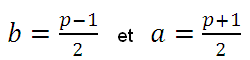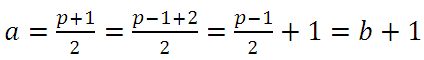### The Exercise Correction 3 natural integers numbers

1) we have for all primes numbers greater than 3 is are odd number , so there is an integer k for p = 2k +1

then :

2) we haveand p is a prime number  greater than 3 ,so there are tow integers numbers a and b deux nombres entiers naturels such asand  :While a and b are consecutive